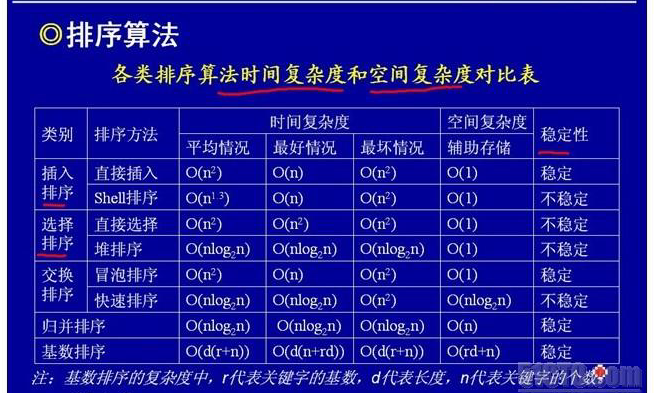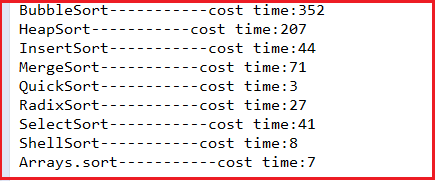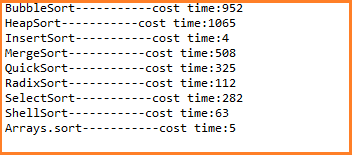# Java 八种排序算法比较实践

写这篇文章是因为面试时经常会问这个问题，但是工作中也没用到过，所以一直是一知半解。但是我是属于比较较真的人，这次下定决心要把它们搞明白。知识在于积累，多点知识对自己总是有好处的。Java Arrays中提供了对所有类型的排序。其中主要分为Primitive（8种基本类型）和Object两大类。

Java对Primitive（int，float等原型数据）数组采用快速排序，对象对象数组采用归并排序。对这一区别，sun在<< Java教程>>中做出的解释如下：

*快速：保证在n log（n）时间内运行，并在几乎排序的列表上运行得更快。经验测试显示它与高度优化的快速排序一样快。快速排序通常被认为比合并排序更快但是不稳定并且不保证n log（n）性能。
*稳定：它不会重新排序相同的元素。如果您对不同的属性重复排序相同的列表，这一点很重要。如果邮件程序的用户通过邮寄日期对收件箱进行排序，然后由发件人对其进行排序，则用户自然希望来自给定发件人的现在连续的邮件列表（仍）按邮件日期排序。只有当第二种类型稳定时才能保证这一点。

也就是说，优化的归并排序既快速（nlog（n））又稳定。
对于对象的排序，稳定性很重要。比如成绩单，一开始可能是按人员的学号顺序排好了的，现在让我们用成绩排，那么你应该保证，本来张三在李四前面，即使他们成绩相同，张三不能跑到李四的后面去。
而快速排序是不稳定的，而且最坏情况下的时间复杂度是O（N ^ 2）。
另外，对象数组中保存的只是对象的引用，这样多次移位并不会造成额外的开销，但是，对象数组对比较次数一般比较敏感，有可能对象的比较比单纯数的比较开销大很多。归并排序在这方面比快速排序做得更好，这也是选择它作为对象排序的一个重要原因之一。

源码中的快速排序，主要做了以下几个方面的优化：
1）当待排序的数组中的元素个数较少时，源码中的阀值为7，采用的是插入排序。尽管插入排序的时间复杂度为0（n ^ 2），但是当数组元素较少时，插入排序优于快速排序，因为这时快速排序的递归操作影响性能
.2）较好的选择了划分元（基准元素） 。能够将数组分成大致两个相等的部分，避免出现最坏的情况。例如当数组有序的的情况下，选择第一个元素作为划分元，将使得算法的时间复杂度达到为O（n ^ 2）。

## 冒泡排序算法：

public	BubbleSort(Integer ar[]){
Integer a[]=ar.clone();
long currentTime=System.currentTimeMillis();
int temp=0;
for(int i=0;i<a.length-1;i++){
for(int j=0;j<a.length-1-i;j++){
if(a[j]>a[j+1]){
temp=a[j];
a[j]=a[j+1];
a[j+1]=temp;
}
}
}

long nowTime=System.currentTimeMillis();
System.out.println("BubbleSort-----------cost time:"+(nowTime-currentTime));
//	System.out.println(Arrays.toString( a));
}
}

## 快速排序算法：

public class QuickSort {
public	QuickSort(Integer ar[]){
Integer a[]=ar.clone();
long currentTime=System.currentTimeMillis();
quick(a);
long nowTime=System.currentTimeMillis();
System.out.println("QuickSort-----------cost time:"+(nowTime-currentTime));
//		System.out.println(Arrays.toString( a));
}
public int getMiddle(Integer[] list, int low, int high) {
int tmp = list[low];    //数组的第一个作为中轴
while (low < high) {
while (low < high && list[high] >= tmp) {

high--;
}
list[low] = list[high];   //比中轴小的记录移到低端
while (low < high && list[low] <= tmp) {
low++;
}
list[high] = list[low];   //比中轴大的记录移到高端
}
list[low] = tmp;              //中轴记录到尾
return low;                   //返回中轴的位置
}
public void _quickSort(Integer[] list, int low, int high) {
if (low < high) {
int middle = getMiddle(list, low, high);  //将list数组进行一分为二
_quickSort(list, low, middle - 1);        //对低字表进行递归排序
_quickSort(list, middle + 1, high);       //对高字表进行递归排序
}
}
public void quick(Integer[] a2) {
if (a2.length > 0) {    //查看数组是否为空
_quickSort(a2, 0, a2.length - 1);
}
}
}

## 归并排序算法：

public class MergeSort {
public	MergeSort(Integer ar[]){
Integer a[]=ar.clone();
long currentTime=System.currentTimeMillis();
sort(a,0,a.length-1);
long nowTime=System.currentTimeMillis();
System.out.println("MergeSort-----------cost time:"+(nowTime-currentTime));
//	System.out.println(Arrays.toString( a));
}
public void sort(Integer[] data, int left, int right) {
// TODO Auto-generated method stub
if(left<right){
//找出中间索引
int center=(left+right)/2;
//对左边数组进行递归
sort(data,left,center);
//对右边数组进行递归
sort(data,center+1,right);
//合并
merge(data,left,center,right);

}
}
public void merge(Integer[] data, int left, int center, int right) {
// TODO Auto-generated method stub
int [] tmpArr=new int[data.length];
int mid=center+1;
//third记录中间数组的索引
int third=left;
int tmp=left;
while(left<=center&&mid<=right){

//从两个数组中取出最小的放入中间数组
if(data[left]<=data[mid]){
tmpArr[third++]=data[left++];
}else{
tmpArr[third++]=data[mid++];
}
}
//剩余部分依次放入中间数组
while(mid<=right){
tmpArr[third++]=data[mid++];
}
while(left<=center){
tmpArr[third++]=data[left++];
}
//将中间数组中的内容复制回原数组
while(tmp<=right){
data[tmp]=tmpArr[tmp++];
}
}

}

## 插入排序算法：

public class InsertSort {
public InsertSort(Integer ar[]){
Integer a[]=ar.clone();
long currentTime=System.currentTimeMillis();
int temp=0;
for(int i=1;i<a.length;i++){
int j=i-1;
temp=a[i];
for(;j>=0&&temp<a[j];j--){
a[j+1]=a[j];                       //将大于temp的值整体后移一个单位
}
a[j+1]=temp;
}
long nowTime=System.currentTimeMillis();
System.out.println("InsertSort-----------cost time:"+(nowTime-currentTime));
//	System.out.println(Arrays.toString( a));
}
}

## 堆排序算法：

public class HeapSort {
public  HeapSort(Integer ar[]){
Integer a[]=ar.clone();
long currentTime=System.currentTimeMillis();
heapSort(a);
long nowTime=System.currentTimeMillis();
System.out.println("HeapSort-----------cost time:"+(nowTime-currentTime));
//		System.out.println(Arrays.toString( a));
}
public  void heapSort(Integer[] a){
int arrayLength=a.length;
//循环建堆
for(int i=0;i<arrayLength-1;i++){
//建堆

buildMaxHeap(a,arrayLength-1-i);
//交换堆顶和最后一个元素
swap(a,0,arrayLength-1-i);

}
}

private  void swap(Integer[] data, int i, int j) {
// TODO Auto-generated method stub
int tmp=data[i];
data[i]=data[j];
data[j]=tmp;
}
//对data数组从0到lastIndex建大顶堆
private void buildMaxHeap(Integer[] data, int lastIndex) {
// TODO Auto-generated method stub
//从lastIndex处节点（最后一个节点）的父节点开始
for(int i=(lastIndex-1)/2;i>=0;i--){
//k保存正在判断的节点
int k=i;
//如果当前k节点的子节点存在
while(k*2+1<=lastIndex){
//k节点的左子节点的索引
int biggerIndex=2*k+1;
//如果biggerIndex小于lastIndex，即biggerIndex+1代表的k节点的右子节点存在
if(biggerIndex<lastIndex){
//若果右子节点的值较大
if(data[biggerIndex]<data[biggerIndex+1]){
//biggerIndex总是记录较大子节点的索引
biggerIndex++;
}
}
//如果k节点的值小于其较大的子节点的值
if(data[k]<data[biggerIndex]){
//交换他们
swap(data,k,biggerIndex);
//将biggerIndex赋予k，开始while循环的下一次循环，重新保证k节点的值大于其左右子节点的值
k=biggerIndex;
}else{
break;
}
}
}
}
}


## 基排序算法：

public RadixSort(Integer ar[]){
Integer a[]=ar.clone();
long currentTime=System.currentTimeMillis();
sort(a);
long nowTime=System.currentTimeMillis();
System.out.println("RadixSort-----------cost time:"+(nowTime-currentTime));
//	System.out.println(Arrays.toString( a));
}
public  void sort(Integer[] array){

//首先确定排序的趟数;
int max=array;
for(int i=1;i<array.length;i++){
if(array[i]>max){
max=array[i];
}
}

int time=0;
//判断位数;
while(max>0){
max/=10;
time++;
}

//建立10个队列;
List<List> queue=new ArrayList<List>();
for(int i=0;i<10;i++){
ArrayList<Integer> queue1=new ArrayList<Integer>();
queue.add(queue1);
}

//进行time次分配和收集;
for(int i=0;i<time;i++){

//分配数组元素;
for(int j=0;j<array.length;j++){
//得到数字的第time+1位数;
int x=array[j]%(int)Math.pow(10, i+1)/(int)Math.pow(10, i);
ArrayList<Integer> queue2=(ArrayList<Integer>) queue.get(x);
queue2.add(array[j]);
queue.set(x, queue2);
}
int count=0;//元素计数器;
//收集队列元素;
for(int k=0;k<10;k++){
while(queue.get(k).size()>0){
ArrayList<Integer> queue3=(ArrayList<Integer>) queue.get(k);
array[count]=queue3.get(0);
queue3.remove(0);
count++;
}
}
}
}

}

## 选择排序算法：

public class SelectSort {
public SelectSort(Integer ar[]){
Integer a[]=ar.clone();
long currentTime=System.currentTimeMillis();
int position=0;
for(int i=0;i<a.length;i++){

int j=i+1;
position=i;
int temp=a[i];
for(;j<a.length;j++){
if(a[j]<temp){
temp=a[j];
position=j;
}
}
a[position]=a[i];
a[i]=temp;
}
long nowTime=System.currentTimeMillis();
System.out.println("SelectSort-----------cost time:"+(nowTime-currentTime));
//		System.out.println(Arrays.toString( a));
}
}

## 希尔排序算法：

public class ShellSort {
public	ShellSort(Integer ar[]){
Integer a[]=ar.clone();
long currentTime=System.currentTimeMillis();
double d1=a.length;
int temp=0;
while(true){
d1= Math.ceil(d1/2);
int d=(int) d1;
for(int x=0;x<d;x++){
for(int i=x+d;i<a.length;i+=d){
int j=i-d;
temp=a[i];
for(;j>=0&&temp<a[j];j-=d){
a[j+d]=a[j];
}
a[j+d]=temp;
}
}
if(d==1)
break;
}
long nowTime=System.currentTimeMillis();
System.out.println("ShellSort-----------cost time:"+(nowTime-currentTime));
//	System.out.println(Arrays.toString( a));
}
}

1.先从数列中取出一个数作为基准数。

2.分区过程，将比这个数大的数全放到它的右边，小于或等于它的数全放到它的左边。

3.再对左右区间重复第二步，直到各区间只有一个数。

 0 1 2 3 4 五 6 7 8 9 72 6 57 88 60 42 83 73 48 85

 0 1 2 3 4 五 6 7 8 9 48 6 57 88 60 42 83 73 88 85

 0 1 2 3 4 五 6 7 8 9 48 6 57 42 60 72 83 73 88 85

1.i = L; j = R; 将基准数挖出形成第一个坑一个[i]中。

2.j--由后向前找比它小的数，找到后挖出此数填前一个坑A 中。

3.I ++由前向后找比它大的数，找到后也挖出此数填到前一个坑一个[J]中。

4.再重复执行2,3-二步，直到我==Ĵ，将基准数填入一个[I]中。

int AdjustArray(int s[], int l, int r) //返回调整后基准数的位置
{
int i = l, j = r;
int x = s[l]; //s[l]即s[i]就是第一个坑
while (i < j)
{
// 从右向左找小于x的数来填s[i]
while(i < j && s[j] >= x)
j--;
if(i < j)
{
s[i] = s[j]; //将s[j]填到s[i]中，s[j]就形成了一个新的坑
i++;
}

// 从左向右找大于或等于x的数来填s[j]
while(i < j && s[i] < x)
i++;
if(i < j)
{
s[j] = s[i]; //将s[i]填到s[j]中，s[i]就形成了一个新的坑
j--;
}
}
//退出时，i等于j。将x填到这个坑中。
s[i] = x;

return i;
}


void quick_sort1(int s[], int l, int r)
{
if (l < r)
{
int i = AdjustArray(s, l, r);//先成挖坑填数法调整s[]
quick_sort1(s, l, i - 1); // 递归调用
quick_sort1(s, i + 1, r);
}
}

//快速排序
void quick_sort(int s[], int l, int r)
{
if (l < r)
{
//Swap(s[l], s[(l + r) / 2]); //将中间的这个数和第一个数交换 参见注1
int i = l, j = r, x = s[l];
while (i < j)
{
while(i < j && s[j] >= x) // 从右向左找第一个小于x的数
j--;
if(i < j)
s[i++] = s[j];

while(i < j && s[i] < x) // 从左向右找第一个大于等于x的数
i++;
if(i < j)
s[j--] = s[i];
}
s[i] = x;
quick_sort(s, l, i - 1); // 递归调用
quick_sort(s, i + 1, r);
}
}

04-15
05-0702-184万+
09-049009
04-282万+
01-0330万+
12-282万+
04-1948
12-171万+
08-191038
10-1365
10-192389
09-136011
10-1466
04-171万+
06-281万+
10-11139
03-082364
03-056536
©️2020 CSDN 皮肤主题: 书香水墨 设计师:CSDN官方博客